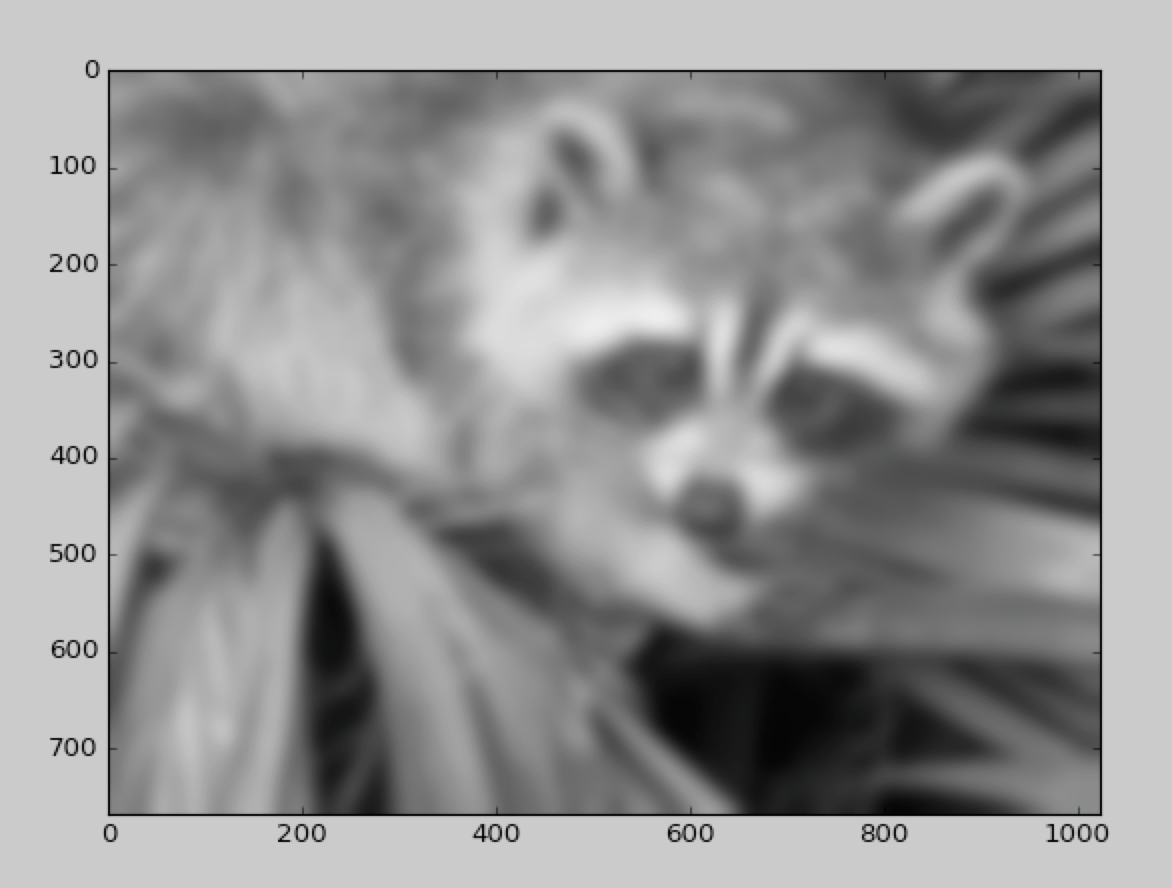# Scientific Python (Scipy) for Machine Learning

1
1707Scientific Python, well known as Scipy is a numerical processing library in Python. It is well known for several pre-built functions for various mathematical tasks that show up often in Machine Learning.

https://www.scipy.org/ is the official website of Scipy. You can download/install Scipy by following the instructions present here: https://www.scipy.org/install.html.

Scipy is open source. https://github.com/scipy/scipy is the main Scipy repository. Scipy comes with a BSD License, which is a free and a permissive license.

Let us now talk about some important applications of Scipy that are often used in Machine Learning and Data Science.

Mathematical Constants

Scipy provides several commonly used Mathematical and Physical Constants. For instance, Scipy provides constants like pi, the speed of light in vacuum, Planck’s Constant, Newton’s Gravitational Constant, Mass of Electron, Avogadro’s Number, etc. Take a look at the following code:

```from scipy.constants import pi, c, h, G, m_e, Avogadro
print "Pi:", pi
print "Speed of light:", c
print "Planck's constant:", h
print "Newton's Gravitational constant:", G
print "Mass of electron:", m_e

The following is the output of the above code:

```Pi: 3.14159265359
Speed of light: 299792458.0
Planck's constant: 6.62607004e-34
Newton's Gravitational constant: 6.67408e-11
Mass of electron: 9.10938356e-31

Besides the above constants, Scipy also provides several unit values in terms of Standard International (SI) units. For instance, take a look at the code below:

```from scipy.constants import gram, day, inch
print "Grams as kgs:", gram
print "Day as seconds:", day
print "Inch in metres:", inch```

The output of this code will be as follows:

```Grams as kgs: 0.001
Day as seconds: 86400.0
Inch in metres: 0.0254```

Importance in Machine Learning: such constants, particularly the mathematical constants often come up in several preprocessing steps in Machine Learning applications.

Fast Fourier Transformation

Fast Fourier Transformation (FFT) is widely used in several Signal Processing applications as a data preprocessing step.

```from scipy.fftpack import fft
import numpy as np
x = np.array([2.1, 3.2, -1.2, 2.0])
print fft(x)```

The output of the above code will be as follows:
[ 6.1+0. j 3.3-1.2j -4.3+0. j 3.3+1.2j]

Numerical Integration

Scipy provides several built-in functions for Numerical Integration as well. For instance, Scipy has functions for Romberg Integration, Simpson’s Rule of Integration, etc. Let us take a look at some examples of how we can perform numerical integration in Scipy:

```import scipy.integrate
f = lambda x: x**2

We get the following output:

(0.33333333333333337, 3.700743415417189e-15)
The first parameter above indicates the value of the integral that Scipy calculated. The second parameter indicates the possible error. As can be seen, the error is of the order of 10-15 which is pretty good given that the actual value of integral is ⅓.

Scipy also provides built-in functions for double integration as well.

Matrices and Determinants

Scipy provides excellent support for solving multivariate linear equations. As an example, take a look at the following:

```from scipy import linalg
import numpy as np

A = np.array([[1, -2, 3], [-1, 3, -1], [2, -5, 5]])
B = np.array([9, -6, 17])

X = linalg.solve(A, B)

print “The Solution is:”, X```

The output of the above code is as follows:

The Solution is: [ 1. -1. 2.]

The above code represents how Scipy can solve a system of the following 3 linear equations:

• x – 2y + 3z = 9
• -x + 3y – z = -16
• 2x – 5y + 5z = 17

As can be seen, Scipy solves it beautifully and is able to get the correct answers.

Similarly, Scipy can find the determinant of several matrices. Take a look at the following code:

```from scipy import linalg
import numpy as np

A = np.array([[1, -2, 3], [-1, 3, -1], [2, -5, 5]])

det = linalg.det(A)

print "The Determinant is:", det```

The output is as follows:

The Determinant is: 1.0

Image Processing

Scipy can perform powerful image manipulations that are often used in the preprocessing step of several Machine Learning applications. As an example, Gaussian blur is one of the most commonly used filters when dealing with Machine Learning applications. Take a look at the code below that demonstrates how Gaussian Filter can be applied to an image in no time using Scipy.

```from scipy import misc, ndimage
import matplotlib.pyplot as plt

image = misc.face()
blurred_image = ndimage.gaussian_filter(image, sigma=10)

plt.imshow(blurred_image)
plt.show()```
```from scipy import misc, ndimage
import matplotlib.pyplot as plt

image = misc.face()
blurred_image = ndimage.gaussian_filter(image, sigma=10)

plt.imshow(blurred_image)
plt.show()```

The output of the above code will be the following image:Finding roots of Mathematical Equations

Scipy provides built-in functions that use the Fixed-point iteration mechanism to find roots of Mathematical Equations. Take a look at the following code:

```from scipy.optimize import root

def my_function(x):
return x**3 + 2

root_of_function = root(my_function, 0.01)
print root_of_function```

The output of the above code will be:

from scipy.optimize import root

def my_function(x):
return x**3 + 2

root_of_function = root(my_function, 0.01)
print root_of_function

The output of the above code will be:

fjac: array([[-1.]])
fun: array([0.])
message: ‘The solution converged.’
nfev: 11
qtf: array([-1.04385389e-10])
r: array([-4.76220423])
status: 1
success: True
x: array([-1.25992105])

Summary

Scipy is a powerful tool and has wide applicability in various applications. You should master this library in order to know how to preprocess data in the best possible way since the preprocessing step often improves the performance of a particular algorithm significantly.

Scipy is most frequently used in combination with other Python libraries like Numpy, Pandas, etc. Numpy is used as an internal “data structure” for holding data of various types in N-dimensional arrays. Pandas, on the other hand, forms a wrapper around Numpy to provide ease of access. You should also have a basic understanding of these libraries in order to get the most out of Scipy.

#### 1 COMMENT

1.blackboxcompetition

Hi! I have saw your blog,I like it very much. Really Informative.Keep sharing Thank You.